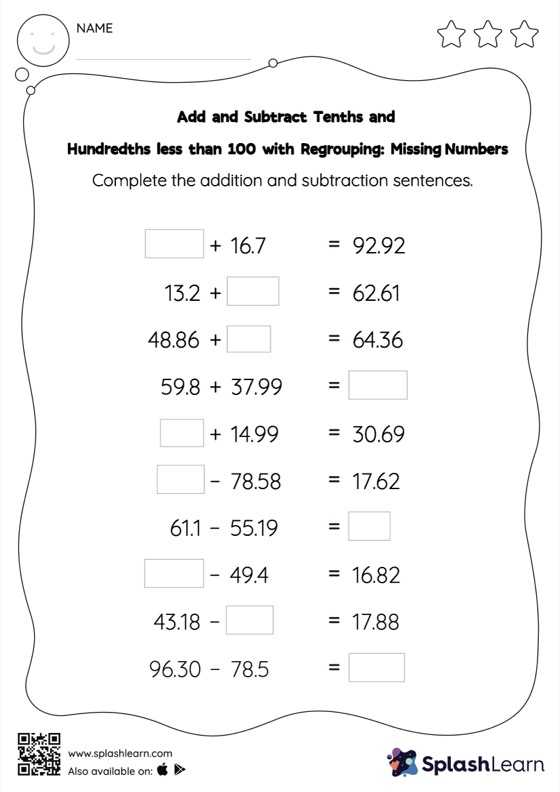# Add and Subtract Tenths and Hundredths less than 100 with Regrouping: Missing Numbers Worksheet

Home > Add and Subtract Tenths and Hundredths less than 100 with Regrouping: Missing NumbersThis worksheet strengthens students' accuracy and strategic flexibility while they add and subtract tenths and hundredths less than 100 with regrouping. Students use the relationship between addition and subtraction to find the missing number while adding or subtracting decimals. To reach the answer, they must also regroup the numbers in add and subtract tenths and hundredths less than 100 with regrouping worksheet using the relationships between ones, tenths, hundredths, thousandths, etc.